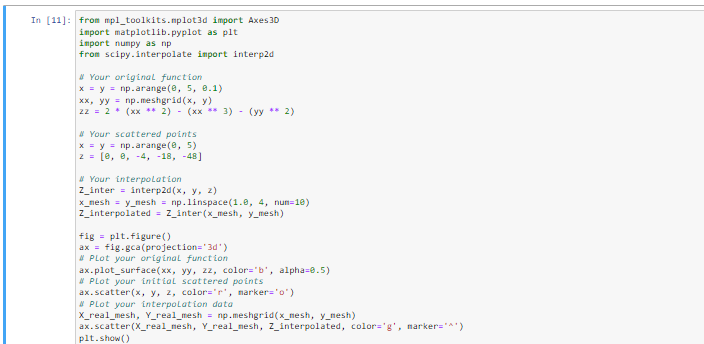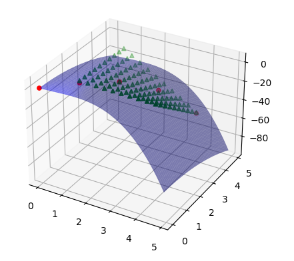# Interpolating a function in two dimensions using SciPy's interp2d functionby sneha@openweaver.comUpdated: Apr 5, 2023Solution Kit

Interpolating a function in two dimensions is approximating a function based on data points in two-dimensional space. It involves finding a function that passes through the given data points and estimating the values of the function between these points.

Scipy is a Python library used for scientific computing. It provides modules for optimization, integration, linear algebra, interpolation, FFT, special functions, ODE solvers, signal and image processing, and other tasks common in science and engineering.

SciPy's interp2d function is a two-dimensional interpolation function that can be used to find the value of a function at a given point in a two-dimensional grid.

• It is useful for plotting data points that are not evenly distributed across the grid.
• It can also be used to interpolate between two surfaces or to fill in missing values in a data set.

Interpolating a function in two dimensions using SciPy's interp2d function is a technique that enables a user to fill in the missing data points in a 2D array by estimating the values of the missing points based on the values of the existing points. The interp2d function uses various interpolation algorithms to generate the missing data points, such as linear, cubic, and higher-order polynomial methods.

Here is an example of Interpolating a function in two dimensions using SciPy's interp2d function.Fig1: Preview of CodeFig2: Preview of the Output

### Code

In this solution, we will use SciPy's interp2d function

### Instructions

1. Install Jupyter Notebook on your computer.
2. Open terminal and install the required libraries with following commands.
3. Install Scipy- pip install scipy
4. Install Numpy - pip install numpy
5. Install matplotlib - pip install matplotlib
6. Copy the snippet using the 'copy' button and paste it into that file.
7. Run the file using run button.

I hope you found this useful. I have added the link to dependent libraries, version information in the following sections.

I found this code snippet by searching for "Interpolating in two dimensions using interp2d" in kandi. You can try any such use case!

### Dependent Libraries﻿

scipyby scipy

Python11340Version:v1.11.0rc1SciPy library main repository

Support
Quality
Security
Reuse

scipyby scipy

Python11340Version:v1.11.0rc1License: Permissive (BSD-3-Clause)

SciPy library main repository
Support
Quality
Security
Reuse

numpyby numpy

Python23755Version:v1.25.0rc1The fundamental package for scientific computing with Python.

Support
Quality
Security
Reuse

numpyby numpy

Python23755Version:v1.25.0rc1License: Permissive (BSD-3-Clause)

The fundamental package for scientific computing with Python.
Support
Quality
Security
Reuse

matplotlibby matplotlib

Python17559Version:v3.7.1License: No License (null)

matplotlib: plotting with Python

Support
Quality
Security
Reuse

matplotlibby matplotlib

Python17559Version:v3.7.1License: No License

matplotlib: plotting with Python
Support
Quality
Security
Reuse

If you do not have SciPy or numpy that is required to run this code, you can install it by clicking on the above link and copying the pip Install command from the numpy page in kandi.

You can search for any dependent library on kandi like numpy/SciPy /matplotlib.

### Environment Tested

I tested this solution in the following versions. Be mindful of changes when working with other versions.

1. The solution is created in Python3.9.6
2. The solution is tested on SciPy-Python 1.9.1 version.

Using this solution, we are able to Interpolate a function in two dimensions using SciPy's interp2d function.

This process also facilities an easy to use, hassle free method to create a hands-on working version of code which would help us to interpolate a function in two dimensions using SciPy's interp2d function.

### Support

1. For any support on kandi solution kits, please use the chat
2. For further learning resources, visit the Open Weaver Community learning page.

See similar Kits and Libraries